# Fraction calculator

The calculator performs basic and advanced operations with fractions, expressions with fractions combined with integers, decimals, and mixed numbers. It also shows detailed step-by-step information about the fraction calculation procedure. Solve problems with two, three, or more fractions and numbers in one expression.

## Result:

### 31/2 - 11/3 = 13/6 = 2 1/6 ≅ 2.1666667

Spelled result in words is thirteen sixths (or two and one sixth).

### How do you solve fractions step by step?

1. Conversion a mixed number 3 1/2 to a improper fraction: 3 1/2 = 3 1/2 = 3 · 2 + 1/2 = 6 + 1/2 = 7/2

To find a new numerator:
a) Multiply the whole number 3 by the denominator 2. Whole number 3 equally 3 * 2/2 = 6/2
b) Add the answer from previous step 6 to the numerator 1. New numerator is 6 + 1 = 7
c) Write a previous answer (new numerator 7) over the denominator 2.

Three and one half is seven halfs
2. Conversion a mixed number 1 1/3 to a improper fraction: 1 1/3 = 1 1/3 = 1 · 3 + 1/3 = 3 + 1/3 = 4/3

To find a new numerator:
a) Multiply the whole number 1 by the denominator 3. Whole number 1 equally 1 * 3/3 = 3/3
b) Add the answer from previous step 3 to the numerator 1. New numerator is 3 + 1 = 4
c) Write a previous answer (new numerator 4) over the denominator 3.

One and one third is four thirds
3. Subtract: 7/2 - 4/3 = 7 · 3/2 · 3 - 4 · 2/3 · 2 = 21/6 - 8/6 = 21 - 8/6 = 13/6
For adding, subtracting, and comparing fractions, it is suitable to adjust both fractions to a common (equal, identical) denominator. The common denominator you can calculate as the least common multiple of both denominators - LCM(2, 3) = 6. In practice, it is enough to find the common denominator (not necessarily the lowest) by multiplying the denominators: 2 × 3 = 6. In the following intermediate step, the fraction result cannot be further simplified by canceling.
In other words - seven halfs minus four thirds = thirteen sixths.

#### Rules for expressions with fractions:

Fractions - use the slash “/” between the numerator and denominator, i.e., for five-hundredths, enter 5/100. If you are using mixed numbers, be sure to leave a single space between the whole and fraction part.
The slash separates the numerator (number above a fraction line) and denominator (number below).

Mixed numerals (mixed fractions or mixed numbers) write as non-zero integer separated by one space and fraction i.e., 1 2/3 (having the same sign). An example of a negative mixed fraction: -5 1/2.
Because slash is both signs for fraction line and division, we recommended use colon (:) as the operator of division fractions i.e., 1/2 : 3.

Decimals (decimal numbers) enter with a decimal point . and they are automatically converted to fractions - i.e. 1.45.

The colon : and slash / is the symbol of division. Can be used to divide mixed numbers 1 2/3 : 4 3/8 or can be used for write complex fractions i.e. 1/2 : 1/3.
An asterisk * or × is the symbol for multiplication.
Plus + is addition, minus sign - is subtraction and ()[] is mathematical parentheses.
The exponentiation/power symbol is ^ - for example: (7/8-4/5)^2 = (7/8-4/5)2

#### Examples:

subtracting fractions: 2/3 - 1/2
multiplying fractions: 7/8 * 3/9
dividing Fractions: 1/2 : 3/4
exponentiation of fraction: 3/5^3
fractional exponents: 16 ^ 1/2
adding fractions and mixed numbers: 8/5 + 6 2/7
dividing integer and fraction: 5 ÷ 1/2
complex fractions: 5/8 : 2 2/3
decimal to fraction: 0.625
Fraction to Decimal: 1/4
Fraction to Percent: 1/8 %
comparing fractions: 1/4 2/3
multiplying a fraction by a whole number: 6 * 3/4
square root of a fraction: sqrt(1/16)
reducing or simplifying the fraction (simplification) - dividing the numerator and denominator of a fraction by the same non-zero number - equivalent fraction: 4/22
expression with brackets: 1/3 * (1/2 - 3 3/8)
compound fraction: 3/4 of 5/7
fractions multiple: 2/3 of 3/5
divide to find the quotient: 3/5 ÷ 2/3

The calculator follows well-known rules for order of operations. The most common mnemonics for remembering this order of operations are:
PEMDAS - Parentheses, Exponents, Multiplication, Division, Addition, Subtraction.
BEDMAS - Brackets, Exponents, Division, Multiplication, Addition, Subtraction
BODMAS - Brackets, Of or Order, Division, Multiplication, Addition, Subtraction.
GEMDAS - Grouping Symbols - brackets (){}, Exponents, Multiplication, Division, Addition, Subtraction.
Be careful, always do multiplication and division before addition and subtraction. Some operators (+ and -) and (* and /) has the same priority and then must evaluate from left to right.

## Fractions in word problems:

• Equation with mixed 2A number, X, is subtracted from 8 1/4. The result is 12 3/5. What is the value of X?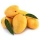A basket contains three types of fruits weighing 87/4 kg in all. If 23/4 kilograms of these are oranges, 48/7 kg are mangoes, and the rest are apples. What is the weight of the apples in the basket?
• Ali bought 2Ali bought 5/6 litre of milk. He drank 1/2 litre and his brother drank 1/6 litre. How much litre of milk left?
• Bucket of clay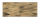Tina and Bill share a 12-ounce bucket of clay. By the end of the week, Tina has used 1/6 of the bucket, and Bill has used 2/3 of the bucket of clay. How many ounces are left in the bucket?
• Mixed numbers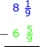Five and two-thirds minus 2 and one-half equals what number? A three and one-sixth B three and two-thirds C three and one-half D three and five-sixths
• SavingsEva borrowed 1/3 of her savings to her brother, 1/2 of savings spent in the store and 7 euros left. How much did she save?
• SundarSundar has 50 chocolates. He gave 2/5 of these chocolates to Ram and he ate 1/5 of them. How many chocolates are left with Sundar?
• Fractions and mixed numerals(a) Convert the following mixed numbers to improper fractions. i. 3 5/8 ii. 7 7/6 (b) Convert the following improper fraction to a mixed number. i. 13/4 ii. 78/5 (c) Simplify these fractions to their lowest terms. i. 36/42 ii. 27/45 2. evaluate the follow
• Benhur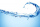Benhur boiled 1 1/4 liters of water in a kettle. After 10 1/2 minutes he measured the water again. It had 3/4 liters left in the kettle. What is the amount of water that evaporates every minutes?
• Square metal sheetWe cut out four squares of 300 mm side from a square sheet metal plate with a side of 0,7 m. Express the fraction and the percentage of waste from the square metal sheet.
• There 17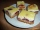There is 3/4 of a cake on a plate in Maria's kitchen.  Silvia sees the cake and eats 1/5 of the cake.  Then Franca takes 1/3 of what was there and shares half of her portion with Antonella.  What fraction of the cake is left?
• From a 2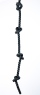From a rope that is 11 m long, two pieces of lengths 13/5 m and 33/10 m are cut off. What is the length of the remaining rope?
• PoundsThree pounds subtract 1/3 of a pound.